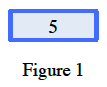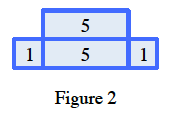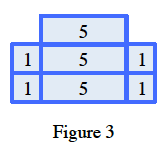### Home > CC3MN > Chapter 5 Unit 5 > Lesson CC3: 5.1.1 > Problem5-5

5-5.

A tile pattern has $5$ tiles in Figure $0$ and adds $7$ tiles in each new figure. Write the equation of the line that represents the growth of this pattern.

Illustrate the problem.

Use the equation of a line to substitute in known information.

$y=mx+b$

$m=$ the rate of growth between each figure
$b=$ the starting number of tiles

$y=7x+5$